## Scikit-Learn, Regression, and PCA, and still more regression.¶

In :
%matplotlib inline
from collections import defaultdict
import json

import numpy as np
import scipy as sp
import matplotlib.pyplot as plt
import pandas as pd

from matplotlib import rcParams
import matplotlib.cm as cm
import matplotlib as mpl

#colorbrewer2 Dark2 qualitative color table
dark2_colors = [(0.10588235294117647, 0.6196078431372549, 0.4666666666666667),
(0.8509803921568627, 0.37254901960784315, 0.00784313725490196),
(0.4588235294117647, 0.4392156862745098, 0.7019607843137254),
(0.9058823529411765, 0.1607843137254902, 0.5411764705882353),
(0.4, 0.6509803921568628, 0.11764705882352941),
(0.9019607843137255, 0.6705882352941176, 0.00784313725490196),
(0.6509803921568628, 0.4627450980392157, 0.11372549019607843)]

rcParams['figure.figsize'] = (10, 6)
rcParams['figure.dpi'] = 150
rcParams['axes.color_cycle'] = dark2_colors
rcParams['lines.linewidth'] = 2
rcParams['axes.facecolor'] = 'white'
rcParams['font.size'] = 14
rcParams['patch.edgecolor'] = 'white'
rcParams['patch.facecolor'] = dark2_colors
rcParams['font.family'] = 'StixGeneral'

def remove_border(axes=None, top=False, right=False, left=True, bottom=True):
"""
Minimize chartjunk by stripping out unnecesasry plot borders and axis ticks

The top/right/left/bottom keywords toggle whether the corresponding plot border is drawn
"""
ax = axes or plt.gca()
ax.spines['top'].set_visible(top)
ax.spines['right'].set_visible(right)
ax.spines['left'].set_visible(left)
ax.spines['bottom'].set_visible(bottom)

#turn off all ticks
ax.yaxis.set_ticks_position('none')
ax.xaxis.set_ticks_position('none')

#now re-enable visibles
if top:
ax.xaxis.tick_top()
if bottom:
ax.xaxis.tick_bottom()
if left:
ax.yaxis.tick_left()
if right:
ax.yaxis.tick_right()

pd.set_option('display.width', 500)
pd.set_option('display.max_columns', 100)

In :
states_abbrev_dict = {
'AL': 'Alabama',
'AR': 'Arkansas',
'AS': 'American Samoa',
'AZ': 'Arizona',
'CA': 'California',
'CT': 'Connecticut',
'DC': 'District of Columbia',
'DE': 'Delaware',
'FL': 'Florida',
'GA': 'Georgia',
'GU': 'Guam',
'HI': 'Hawaii',
'IA': 'Iowa',
'ID': 'Idaho',
'IL': 'Illinois',
'IN': 'Indiana',
'KS': 'Kansas',
'KY': 'Kentucky',
'LA': 'Louisiana',
'MA': 'Massachusetts',
'MD': 'Maryland',
'ME': 'Maine',
'MI': 'Michigan',
'MN': 'Minnesota',
'MO': 'Missouri',
'MP': 'Northern Mariana Islands',
'MS': 'Mississippi',
'MT': 'Montana',
'NA': 'National',
'NC': 'North Carolina',
'ND': 'North Dakota',
'NH': 'New Hampshire',
'NJ': 'New Jersey',
'NM': 'New Mexico',
'NY': 'New York',
'OH': 'Ohio',
'OK': 'Oklahoma',
'OR': 'Oregon',
'PA': 'Pennsylvania',
'PR': 'Puerto Rico',
'RI': 'Rhode Island',
'SC': 'South Carolina',
'SD': 'South Dakota',
'TN': 'Tennessee',
'TX': 'Texas',
'UT': 'Utah',
'VA': 'Virginia',
'VI': 'Virgin Islands',
'VT': 'Vermont',
'WA': 'Washington',
'WI': 'Wisconsin',
'WV': 'West Virginia',
'WY': 'Wyoming'
}
abbrev_states_dict = {v: k for k, v in states_abbrev_dict.items()}


### First, a bit about scikit-learn¶

The structure of scikit-learn:

Some of the following text is taken from the scikit-learn API paper: http://arxiv.org/pdf/1309.0238v1.pdf

All objects within scikit-learn share a uniform common basic API consisting of three complementary interfaces: an estimator interface for building and ﬁtting models, a predictor interface for making predictions and a transformer interface for converting data.

The estimator interface is at the core of the library. It deﬁnes instantiation mechanisms of objects and exposes a fit method for learning a model from training data. All supervised and unsupervised learning algorithms (e.g., for classiﬁcation, regression or clustering) are oﬀered as objects implementing this interface. Machine learning tasks like feature extraction, feature selection or dimensionality reduction are also provided as estimators.

An example along these lines:

clf = LogisticRegression()
clf.fit(X_train, y_train)



If one changes classifiers, say, to a Random Forest classifier, one would simply replace LogisticRegression() in the snippet above by RandomForestClassifier().

The predictor interface extends the notion of an estimator by adding a predict method that takes an array X_test and produces predictions for X_test, based on the learned parameters of the estimator. In the case of supervised learning estimators, this method typically returns the predicted labels or values computed by the model. Some unsupervised learning estimators may also implement the predict interface, such as k-means, where the predicted values are the cluster labels.

clf.predict(X_test)

Since it is common to modify or ﬁlter data before feeding it to a learning algorithm, some estimators in the library implement a transformer interface which deﬁnes a transform method. It takes as input some new data X_test and yields as output a transformed version of X_test. Preprocessing, feature selection, feature extraction and dimensionality reduction algorithms are all provided as transformers within the library.

This is usually done via the fit_transform method. For example, to do a PCA:

pca = RandomizedPCA(n_components=2)
train_x = pca.fit_transform(train_x)
test_x = pca.transform(test_x)



The training set here is "fit" to find the PC components, and then then transformed. Since pca.fit() by itself changes the pca object, if we want to transform other data using the same transformation we simply call transform subsequently.

Finally, for now, there is the concept of a meta-estimator, which behaves quite similarly to standard estimators, but allows us to wrap, for example, cross-validation, or methods that build and combine simpler models or schemes. For example:

from sklearn.multiclass import OneVsOneClassifier
clf=OneVsOneClassifier(LogisticRegression())



In scikit-learn, model selection is supported in two distinct meta-estimators, GridSearchCV and RandomizedSearchCV. They take as input an estimator (basic or composite), whose hyper-parameters must be optimized, and a set of hyperparameter settings to search through.

We shall use this latter functionality soon (and you have seen an example of it in HW2).

Most machine learning algorithms implemented in scikit-learn expect data to be stored in a two-dimensional array or matrix. The arrays can be either numpy arrays, or in some cases scipy.sparse matrices. The size of the array is expected to be [n_samples, n_features]

To get a grip on how to do machine learning with scikit-learn, it is worth working through the entire set of notebooks at: https://github.com/jakevdp/sklearn_pycon2013 . These go relatively fast, are fun to read. The repository at https://github.com/jakevdp/sklearn_scipy2013 has more advanced notebooks. The rendered_notebooks folder here is useful with worked-out examples.

### PART 1: Linear Regression with a touch of PCA.¶

We'll see an example of the concepts mentioned above by considering a linear regression problem. Let us load the census data set.

In :
census_data = pd.read_csv("./data/census_demographics.csv")

Out:
state per_black per_hisp per_white educ_hs educ_coll average_income median_income pop_density vote_pop older_pop per_older per_vote
0 ALABAMA 26.5 4.0 66.8 81.4 21.7 22984 42081 94.4 3001712.500 672383.600 0.140 0.625
1 ALASKA 3.6 5.8 63.7 90.7 27.0 30726 66521 1.2 475548.444 58540.158 0.081 0.658
2 ARIZONA 4.5 30.1 57.4 85.0 26.3 25680 50448 56.3 3934880.535 920515.710 0.142 0.607
3 ARKANSAS 15.6 6.6 74.2 81.9 19.1 21274 39267 56.0 1798043.148 428944.934 0.146 0.612
4 CALIFORNIA 6.6 38.1 39.7 80.7 30.1 29188 60883 239.1 24009747.944 4409953.704 0.117 0.637

Clean the data set, and have it indexed by the state abbrev.

In :
def capitalize(s):
s = s.title()
s = s.replace("Of", "of")
return s
census_data["State"] = census_data.state.map(capitalize)
del census_data["state"]
census_data['State']=census_data['State'].replace(abbrev_states_dict)
census_data.set_index("State", inplace=True)

Out:
per_black per_hisp per_white educ_hs educ_coll average_income median_income pop_density vote_pop older_pop per_older per_vote
State
AL 26.5 4.0 66.8 81.4 21.7 22984 42081 94.4 3001712.500 672383.600 0.140 0.625
AK 3.6 5.8 63.7 90.7 27.0 30726 66521 1.2 475548.444 58540.158 0.081 0.658
AZ 4.5 30.1 57.4 85.0 26.3 25680 50448 56.3 3934880.535 920515.710 0.142 0.607
AR 15.6 6.6 74.2 81.9 19.1 21274 39267 56.0 1798043.148 428944.934 0.146 0.612
CA 6.6 38.1 39.7 80.7 30.1 29188 60883 239.1 24009747.944 4409953.704 0.117 0.637

We use a SPLOM to visualize some columns of this dataset. In Panda's the SPLOM is a one-liner.

In :
smaller_frame=census_data[['educ_coll', 'average_income', 'per_vote']]
from pandas.tools.plotting import scatter_matrix
axeslist=scatter_matrix(smaller_frame, alpha=0.8, figsize=(12, 12), diagonal="kde")
for ax in axeslist.flatten():
ax.grid(False)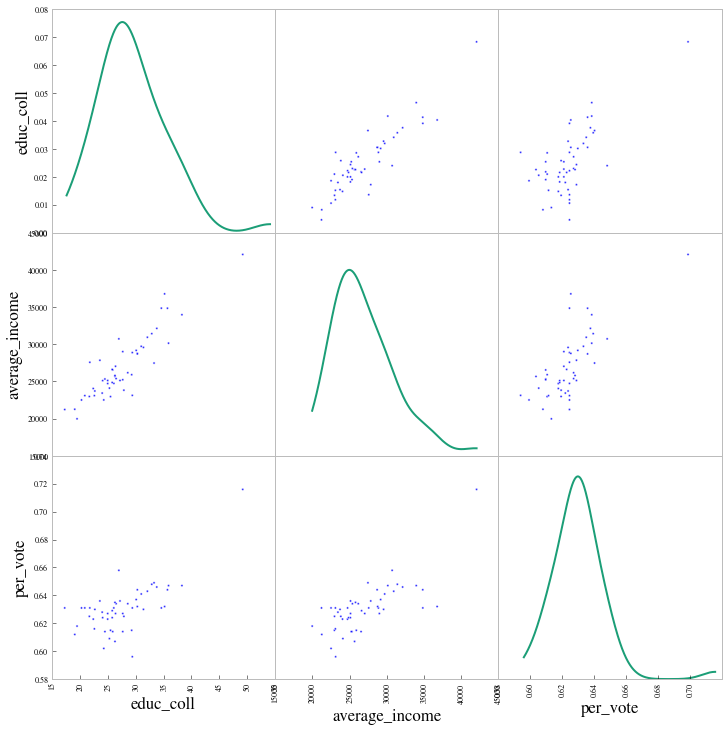Notice how average_income seems to have a strong correlation with educ_coll. Lets try and regress the former against the latter. One might expect that the average income is higher in states which have "better" education systems and send more students to college. First lets confirm our intuition by seeing the co-relations.

In :
smaller_frame.corr()

Out:
educ_coll average_income per_vote
educ_coll 1.000000 0.894066 0.670977
average_income 0.894066 1.000000 0.732703
per_vote 0.670977 0.732703 1.000000

We carry out the regression, first standardizing our variables. This is strictly not necessary, but we are doing it as we wish to play around with PCA. Since scikit-learn wants a n_sample rows times n_features matrix, we need to reshape the x variable. We store both an _vec variable, which is easier to plot with, as well as the reshaped variable.

In :
from sklearn.linear_model import LinearRegression
X_HD=smaller_frame[['educ_coll', 'average_income']].values
X_HDn=(X_HD - X_HD.mean(axis=0))/X_HD.std(axis=0)
educ_coll_std_vec=X_HDn[:,0]
educ_coll_std=educ_coll_std_vec.reshape(-1,1)
average_income_std_vec=X_HDn[:,1]
average_income_std=average_income_std_vec.reshape(-1,1)


We split the data into a training set and a testing set. By default, 25% of the data is reserved for testing. This is the first of multiple ways that we will see to do this.

In :
from sklearn.cross_validation import train_test_split
X_train, X_test, y_train, y_test = train_test_split(educ_coll_std, average_income_std_vec)


We use the training set for the fit, and find what our predictions ought to be on both the training and test set.

In :
clf1 = LinearRegression()
clf1.fit(X_train, y_train)
predicted_train = clf1.predict(X_train)
predicted_test = clf1.predict(X_test)
trains=X_train.reshape(1,-1).flatten()
tests=X_test.reshape(1,-1).flatten()
print clf1.coef_, clf1.intercept_

[ 0.92412128] -0.00156831593755


We plot the scatter against the fit for both training and test data.

In :
plt.scatter(educ_coll_std_vec, average_income_std_vec,c='r')
plt.plot(trains, predicted_train, c='g', alpha=0.5)
plt.plot(tests, predicted_test, c='g', alpha=0.2)

Out:
[<matplotlib.lines.Line2D at 0x10859f790>]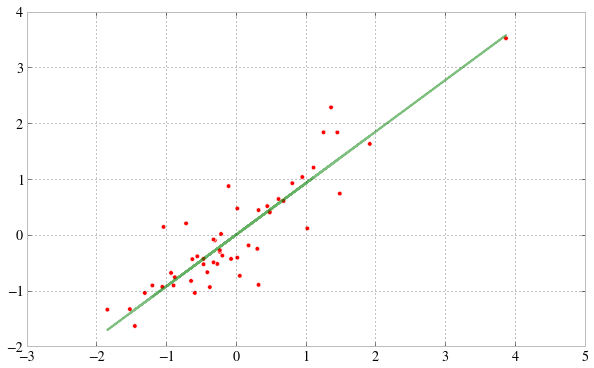We then look at the residuals, again on both sets.

In :
plt.scatter(predicted_test, predicted_test- y_test, c='g', s=40)
plt.scatter(predicted_train, predicted_train- y_train, c='b', s=40, alpha=0.5)
plt.plot([0.4,2],[0,0])

Out:
[<matplotlib.lines.Line2D at 0x1085d4450>]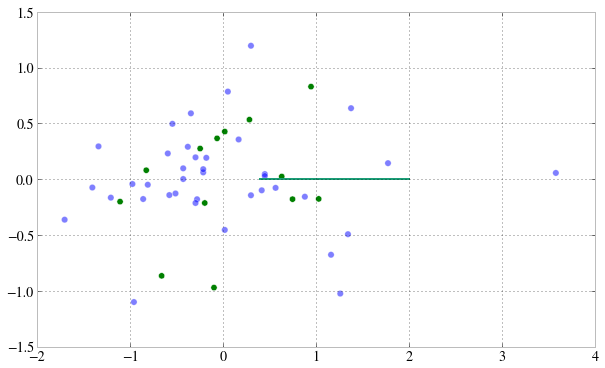We ask scikit-learn to spit out the $R^2$. If you'd like R-style detailed information about your regression, use statsmodels instead.

In :
clf1.score(X_train, y_train), clf1.score(X_test, y_test)

Out:
(0.84357153741408697, 0.44645202007349571)

#### Doing a PCA on the data¶

Lets take the standarddized data and do a 2-D PCA on it. Here we do not seek to accomplish a dimensional reduction, but to understand the variance structure of the data.

In :
from sklearn.decomposition import PCA
pca = PCA(n_components=2)
X = pca.fit_transform(X_HDn)
print pca.explained_variance_ratio_

[ 0.94703283  0.05296717]

In :
plt.scatter(X[:, 0], X[:, 1])

Out:
<matplotlib.collections.PathCollection at 0x108b72550>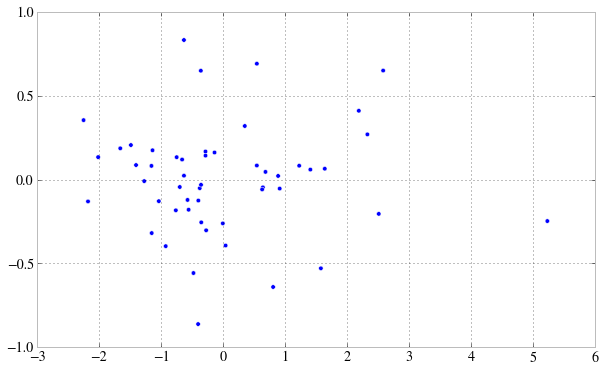Since the first component is so latge, lets only keep it, and then reconstruct the original data from only this component, setting the others to 0.

In :
pca1 = PCA(n_components=1) # only keep one dimension!
X_E = pca1.fit_transform(X_HDn)
X_reconstructed = pca1.inverse_transform(X_E)


We plot the reconstructed education(x) and income(y) from the first principal component, in blue.

In :
plt.scatter(X_reconstructed[:,0], X_reconstructed[:,1],c='b', s=35, alpha=0.7)
plt.scatter(educ_coll_std_vec, average_income_std_vec, s=40, c='r', alpha=0.6)
plt.plot(trains, predicted_train, c='g', alpha=0.3)
plt.plot(tests, predicted_test, c='g', alpha=0.3)

Out:
[<matplotlib.lines.Line2D at 0x108c80850>]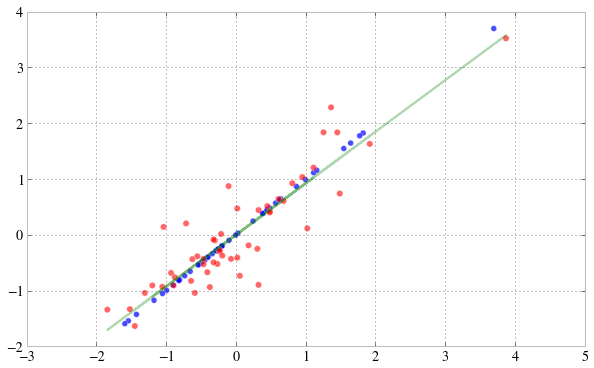We see that the principal component line is steeper as we saw in class. The principle behind the projections is captured below in this plot from stack overflow that HP showed in class.Regress the variables in the other direction: ie the educ_coll against the average_income. This might feel strange to you but remember regression is not about CAUSALITY, only about CORRELATION.

In :
#your code here
clf2 = LinearRegression()
clf2.fit(average_income_std, educ_coll_std_vec)
print clf2.coef_,clf2.intercept_

[ 0.89406566] 1.99131419306e-15


Create a similar scatterplot and fit line for this regression.

In :
plt.plot(average_income_std_vec, clf2.predict(average_income_std))
plt.scatter(average_income_std_vec, educ_coll_std_vec)

Out:
<matplotlib.collections.PathCollection at 0x108b7e290>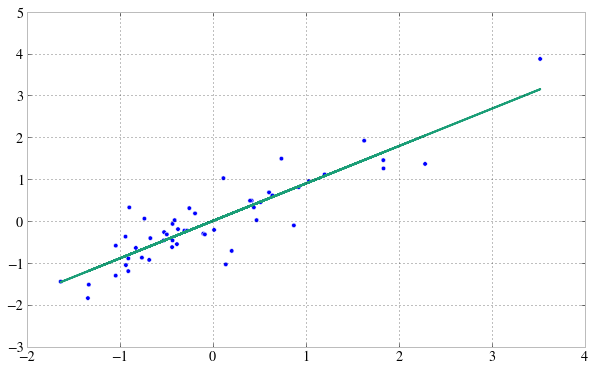### Part 2: Logistic Regression¶

From http://www.edwardtufte.com/tufte/ebooks, in "Visual and Statistical Thinking: Displays of Evidence for Making Decisions":

On January 28, 1986, the space shuttle Challenger exploded and seven astronauts died because two rubber O-rings leaked. These rings had lost their resiliency because the shuttle was launched on a very cold day. Ambient temperatures were in the low 30s and the O-rings themselves were much colder, less than 20F.

One day before the flight, the predicted temperature for the launch was 26F to 29F. Concerned that the rings would not seal at such a cold temperature, the engineers who designed the rocket opposed launching Challenger the next day.

But they did not make their case persuasively, and were over-ruled by NASA.

In :
from IPython.display import Image as Im
from IPython.display import display
Im('./data/shuttle.png')

Out: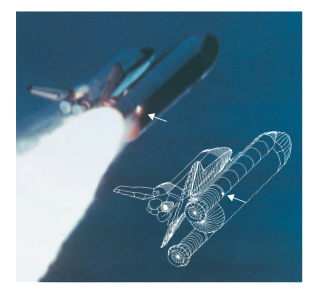The image above shows the leak, where the O-ring failed.

We have here data on previous failures of the O-rings at various temperatures.

In :
data=np.array([[float(j) for j in e.strip().split()] for e in open("./data/chall.txt")])
data

Out:
array([[ 66.,   0.],
[ 70.,   1.],
[ 69.,   0.],
[ 68.,   0.],
[ 67.,   0.],
[ 72.,   0.],
[ 73.,   0.],
[ 70.,   0.],
[ 57.,   1.],
[ 63.,   1.],
[ 70.,   1.],
[ 78.,   0.],
[ 67.,   0.],
[ 53.,   1.],
[ 67.,   0.],
[ 75.,   0.],
[ 70.,   0.],
[ 81.,   0.],
[ 76.,   0.],
[ 79.,   0.],
[ 75.,   1.],
[ 76.,   0.],
[ 58.,   1.]])

Lets plot this data

In :
temps, pfail = data[:,0], data[:,1]
plt.scatter(temps, pfail)
axes=plt.gca()
axes.grid(False)
remove_border(axes)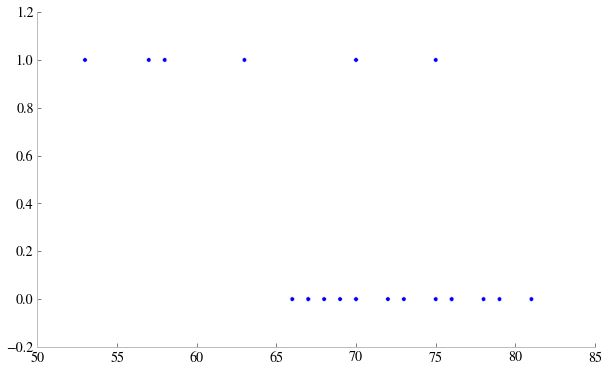1 represents failure. This graph has a classic sigmoid shape, so one might expect logistic regression to work. Furthermore, we do want to find the probability of failure and make predictions from there.

Logistic regression is carried out in the same way as linear. However, there is the "pesky" matter of setting the regularization co-efficient C. We havent still covered this in class, so what we do today is just a preview, but its nonetheless important to see.

The default C in sklearn is 1.The meaning of C is: the larger the C, the lesser the regularization. The smaller the C the higher the regularization.

What does regularization do? Larger regularizations penalize the values of regression coefficients. Smaller ones let the co-efficients range widely. Thus, larger C let the regression coefficients range widely. Scikit-learn bakes in two penalties: a l2 penalty which penalizes the sum of the squares of the coefficients, and a l1 penalty which penalizes the sum of the absolute values.

The reason for doing this is that is there are many co-variates we use for our prediction: we want to make sure we can get away with the simplest model that describes our data, even if that might increase the bias side of the bias-variance tradeoff a bit.

Remember here, though, that we have just two co-efficents: an intercept, and the outside temperature. So we do not expect to need regularization much. Indeed lets set C=1000.

In :
from sklearn.linear_model import LogisticRegression
reg=1000.
clf4 = LogisticRegression(C=reg)
clf4.fit(temps.reshape(-1,1), pfail)

Out:
LogisticRegression(C=1000.0, class_weight=None, dual=False,
fit_intercept=True, intercept_scaling=1, penalty='l2',
random_state=None, tol=0.0001)

Lets make predictions, get the associated probabilities, and plot them. We create a new grid of temperatures to evaluate our predictions at. Note that we do not do a test/train split: we have only 23 data points, but need to shut down the launch if there is any doubt. (One wishes...)

In :
tempsnew=np.linspace(20., 90., 15)
probs = clf4.predict_proba(tempsnew.reshape(-1,1))[:, 1]
predicts = clf4.predict(tempsnew.reshape(-1,1))

In :
plt.scatter(temps, pfail)
axes=plt.gca()
axes.grid(False)
remove_border(axes)
plt.plot(tempsnew, probs, marker='s')
plt.scatter(tempsnew, predicts, marker='s', color="green")

Out:
<matplotlib.collections.PathCollection at 0x108cd3350>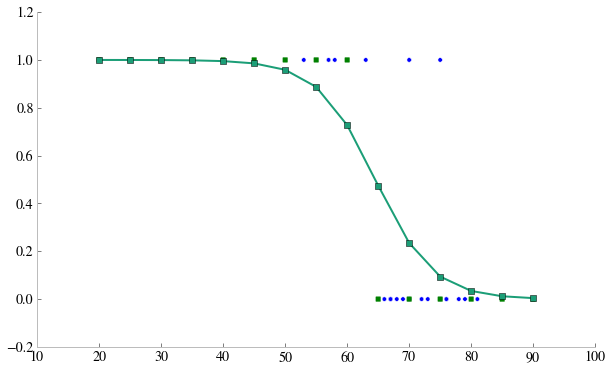We use pandas crosstab to write a table of prediction vs failure on the "training" set. As one might expect, the mislabellings come at the higher temperatures.

In :
pd.crosstab(pfail, clf4.predict(temps.reshape(-1,1)), rownames=["Actual"], colnames=["Predicted"])

Out:
Predicted 0.0 1.0
Actual
0 16 0
1 3 4

Carry out a Logistic Regression with scikit-learn's default value for C. Make a plot similar to the scatterplot above, and carry out the cross-tabulation. What happens?

In :
#your code here
clf4w = LogisticRegression()
clf4w.fit(temps.reshape(-1,1), pfail)
probsw = clf4w.predict_proba(tempsnew.reshape(-1,1))[:, 1]
predictsw = clf4w.predict(tempsnew.reshape(-1,1))
plt.scatter(temps, pfail)
axes=plt.gca()
axes.grid(False)
remove_border(axes)
plt.plot(tempsnew, probsw, marker='s')
plt.scatter(tempsnew, predictsw, marker='s', color="green")

Out:
<matplotlib.collections.PathCollection at 0x1091df350>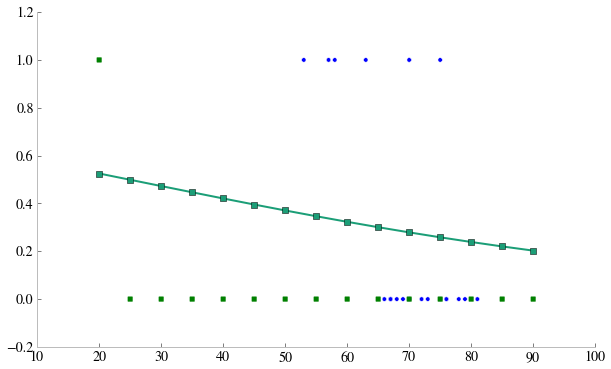In :
#your code here
pd.crosstab(pfail, clf4w.predict(temps.reshape(-1,1)), rownames=["Actual"], colnames=["Predicted"])

Out:
Predicted 0.0
Actual
0 16
1 7

#### Logistic Regression with cross-validation¶

We now actually go ahead and to the train/test split. Not once but multiple times, on a grid search, for different values of C. For each C, we:

1. create n_folds folds. Since the data size is 23 here, and we have 5 folds, we roughly split the data into 5 folds of 4-5 values each, randomly.
2. We then train on 4 of these folds, test on the 5th
3. We average the results of all such combinations
4. We move on to the next value of C, and find the optimal value that minimizes mean square error.
5. We finally use that value to make the final fit.

Notice the structure of the GridSearchCV estimator in cv_optimize.

In :
from sklearn.linear_model import LogisticRegression
def fit_logistic(X_train, y_train, reg=0.0001, penalty="l2"):
clf = LogisticRegression(C=reg, penalty=penalty)
clf.fit(X_train, y_train)
return clf

from sklearn.grid_search import GridSearchCV

def cv_optimize(X_train, y_train, paramslist, penalty="l2", n_folds=10):
clf = LogisticRegression(penalty=penalty)
parameters = {"C": paramslist}
gs = GridSearchCV(clf, param_grid=parameters, cv=n_folds)
gs.fit(X_train, y_train)
return gs.best_params_, gs.best_score_

def cv_and_fit(X_train, y_train, paramslist, penalty="l2", n_folds=5):
bp, bs = cv_optimize(X_train, y_train, paramslist, penalty=penalty, n_folds=n_folds)
print "BP,BS", bp, bs
clf = fit_logistic(X_train, y_train, penalty=penalty, reg=bp['C'])
return clf

In :
clf=cv_and_fit(temps.reshape(-1,1), pfail, np.logspace(-4, 3, num=100))

BP,BS {'C': 521.40082879996839} 0.87

In :
pd.crosstab(pfail, clf.predict(temps.reshape(-1,1)), rownames=["Actual"], colnames=["Predicted"])

Out:
Predicted 0.0 1.0
Actual
0 16 0
1 3 4

We plot our results, this time also marking in red the predictions on the "training" set.

In :
plt.scatter(temps, pfail, s=40)
axes=plt.gca()
axes.grid(False)
remove_border(axes)
probs=clf.predict_proba(tempsnew.reshape(-1,1))[:,1]
predicts=clf.predict(tempsnew.reshape(-1,1))
plt.plot(tempsnew, probs, marker='s')
plt.scatter(tempsnew, predicts, marker='D', color="green", s=80, alpha=0.4)
train_probs=clf.predict_proba(temps.reshape(-1,1))[:,1]
plt.scatter(temps, train_probs, marker='s', c='r', alpha=0.5, s=40)
train_predicts=clf.predict(temps.reshape(-1,1))
plt.scatter(temps, train_predicts, marker='s', c='r', alpha=0.2, s=80)

Out:
<matplotlib.collections.PathCollection at 0x10922ac10>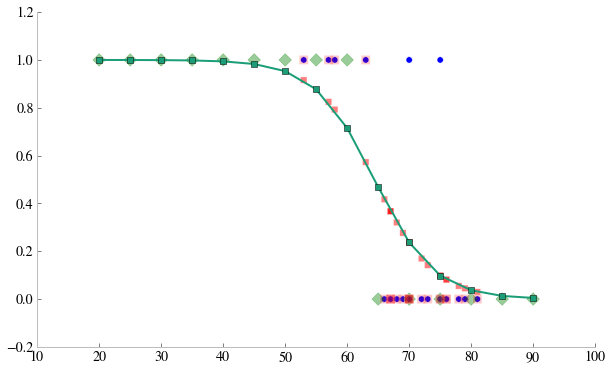The failures in prediction are, exactly where you might have expected them to be, as before.

In :
zip(temps,pfail, clf.predict(temps.reshape(-1,1)))

Out:
[(66.0, 0.0, 0.0),
(70.0, 1.0, 0.0),
(69.0, 0.0, 0.0),
(68.0, 0.0, 0.0),
(67.0, 0.0, 0.0),
(72.0, 0.0, 0.0),
(73.0, 0.0, 0.0),
(70.0, 0.0, 0.0),
(57.0, 1.0, 1.0),
(63.0, 1.0, 1.0),
(70.0, 1.0, 0.0),
(78.0, 0.0, 0.0),
(67.0, 0.0, 0.0),
(53.0, 1.0, 1.0),
(67.0, 0.0, 0.0),
(75.0, 0.0, 0.0),
(70.0, 0.0, 0.0),
(81.0, 0.0, 0.0),
(76.0, 0.0, 0.0),
(79.0, 0.0, 0.0),
(75.0, 1.0, 0.0),
(76.0, 0.0, 0.0),
(58.0, 1.0, 1.0)]

We note that the true story was even worse than our data made it out to be! We did not take the severity of the incidents into account. How could we have incorporated this severity into our analysis? (these images are taken from Tufte's booklet).

In :
Im('./data/chall-table.png')

Out: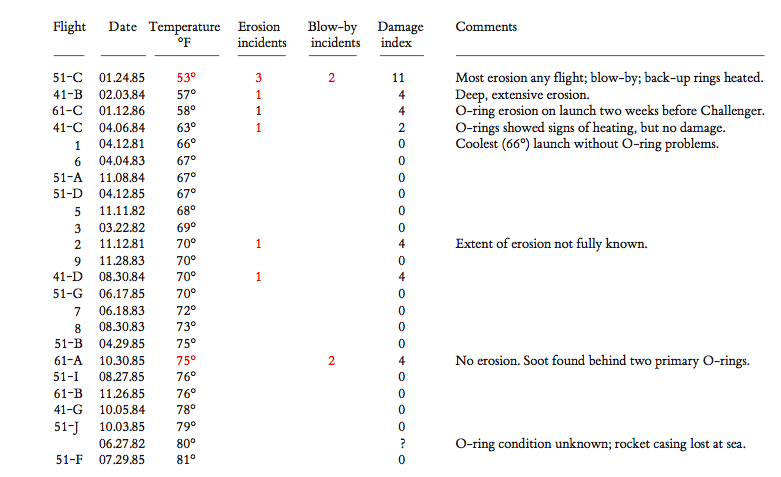### Part 3: PCA¶

In :
from PIL import Image


You are an ATM and have to distinguish between cash and check. Its based on a check/drivers license separator at the yhat blog (http://blog.yhathq.com/posts/image-classification-in-Python.html), and a fair bit of code is obtained from there. This problem is a bit more interesting as there is more structure in the images.

We standardize the size of the images:

In :
#setup a standard image size; this will distort some images but will get everything into the same shape
STANDARD_SIZE = (322, 137)
def img_to_matrix(filename, verbose=False):
"""
takes a filename and turns it into a numpy array of RGB pixels
"""
img = Image.open(filename)
if verbose==True:
print "changing size from %s to %s" % (str(img.size), str(STANDARD_SIZE))
img = img.resize(STANDARD_SIZE)
img = list(img.getdata())
img = map(list, img)
img = np.array(img)
return img

def flatten_image(img):
"""
takes in an (m, n) numpy array and flattens it
into an array of shape (1, m * n)
"""
s = img.shape * img.shape
img_wide = img.reshape(1, s)
return img_wide

In :
import os
checks_dir = "./data/images/images/checks/"
dollars_dir = "./data/images/images/dollars/"
def images(img_dir):
return [img_dir+f for f in os.listdir(img_dir)]
checks=images(checks_dir)
dollars=images(dollars_dir)
images=checks+dollars
labels = ["check" for i in range(len(checks))] + ["dollar" for i in range(len(dollars))]
len(labels), len(images)

Out:
(87, 87)

Lets see what some of these images look like:

In :
for i in range(3):
display(Im(checks[i]))
for i in range(3):
display(Im(dollars[i]))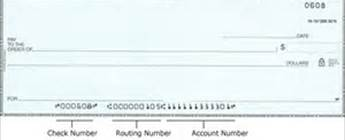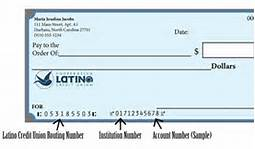What features might you use to istinguish the notes from the checks?

Here is an example of transforming an image into its R channel.

In :
i0=images
display(Im(i0))
i0m=img_to_matrix(i0)
print i0m.shape
plt.imshow(i0m[:,1].reshape(137,322))(44114, 3)

Out:
<matplotlib.image.AxesImage at 0x10a81ab10>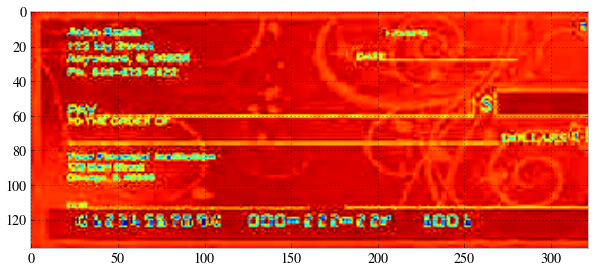We do this for every image, flattening each image into 3 channels of 44114 pixels, for a total of 132342 features per image!

In :
data = []
for image in images:
img = img_to_matrix(image)
img = flatten_image(img)
data.append(img)

data = np.array(data)
data.shape

Out:
(87, 132342)
In :
y =</